## Adding Fractions With Common Denominators

### Learning Outcomes

• Use a model to find the sum of two fractions with the same denominator
• Add fractions with a common denominator without a model
• Add fractions with a common denominator that contain a variable

How many quarters are pictured? One quarter plus $2$ quarters equals $3$ quarters.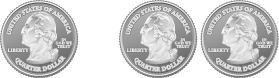Remember, quarters are really fractions of a dollar. Quarters are another way to say fourths. So the picture of the coins shows that

${\Large\frac{1}{4}}+{\Large\frac{2}{4}}=\Large{\frac{3}{4}}$

$\text{one quarter }+\text{ two quarters }=\text{ three quarters}$

Let’s use fraction circles to model the same example, $\Large\frac{1}{4}\normalsize+\Large\frac{2}{4}$.

 Start with one $\Large\frac{1}{4}$ piece.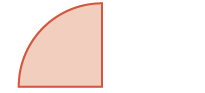$\Large\frac{1}{4}$ Add two more $\Large\frac{1}{4}$ pieces.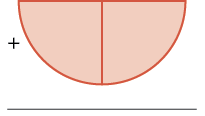$+\Large\frac{2}{4}$ The result is $\Large\frac{3}{4}$ .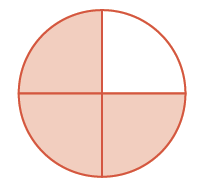$\Large\frac{3}{4}$

So again, we see that

$\Large\frac{1}{4}\normalsize+\Large\frac{2}{4}\normalsize=\Large\frac{3}{4}$

### example

Use a model to find the sum $\Large\frac{3}{8}\normalsize+\Large\frac{2}{8}$.

Solution:

 Start with three $\Large\frac{1}{8}$ pieces.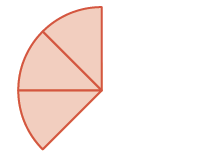$\Large\frac{3}{8}$ Add two $\Large\frac{1}{8}$ pieces.$+\Large\frac{2}{8}$ How many $\Large\frac{1}{8}$ pieces are there?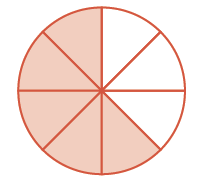$\Large\frac{5}{8}$

There are five $\Large\frac{1}{8}$ pieces, or five-eighths. The model shows that $\Large\frac{3}{8}\normalsize+\Large\frac{2}{8}\normalsize=\Large\frac{5}{8}$.

### try it

Use a model to find each sum. Show a diagram to illustrate your model.

$\Large\frac{1}{8}\normalsize+\Large\frac{4}{8}$

Use a model to find each sum. Show a diagram to illustrate your model.
$\Large\frac{1}{6}\normalsize+\Large\frac{4}{6}$

The following video shows more examples of how to use models to add fractions with like denominators.

## Add Fractions with a Common Denominator

The example above shows that to add the same-size pieces—meaning that the fractions have the same denominator—we just add the number of pieces.

If $a,b,\text{ and }c$ are numbers where $c\ne 0$, then

$\Large\frac{a}{c}\normalsize+\Large\frac{b}{c}\normalsize=\Large\frac{a+b}{c}$
To add fractions with a common denominators, add the numerators and place the sum over the common denominator.

### Example

Find the sum: $\Large\frac{3}{5}\normalsize+\Large\frac{1}{5}$

### Example

Find the sum: $\Large\frac{x}{3}\normalsize+\Large\frac{2}{3}$

Note that we cannot simplify this fraction any more. Since $x\text{ and }2$ are not like terms, we cannot combine them.

### Example

Find the sum: $-\Large\frac{9}{d}\normalsize+\Large\frac{3}{d}$

### Example

Find the sum: $\Large\frac{2n}{11}\normalsize+\Large\frac{5n}{11}$

### Example

Find the sum: $\Large-\frac{3}{12}+\left(-\frac{5}{12}\right)$

### Try It

in the next video we show three more examples of adding fractions with common denominators. Pay close attention to the second example, it addresses a common mistake made by students when simplifying fractions with variables.